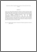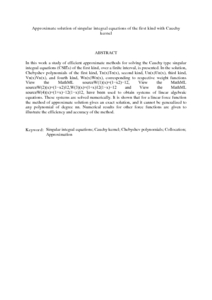Simple Search:

Approximate solution of singular integral equations of the first kind with Cauchy kernel

Citation

Eshkuratov, Zainidin K. and Nik Long, Nik Mohd. Asri and Abdulkawi, M. (2009) Approximate solution of singular integral equations of the first kind with Cauchy kernel. Applied Mathematics Letters, 22 (5 ). pp. 651-657. ISSN 0893-9659; ESSN: 1873-5452

Abstract / Synopsis

In this work a study of efficient approximate methods for solving the Cauchy type singular integral equations (CSIEs) of the first kind, over a finite interval, is presented. In the solution, Chebyshev polynomials of the first kind, Tn(x)Tn(x), second kind, Un(x)Un(x), third kind, Vn(x)Vn(x), and fourth kind, Wn(x)Wn(x), corresponding to respective weight functions View the MathML sourceW(1)(x)=(1−x2)−12, View the MathML sourceW(2)(x)=(1−x2)12,W(3)(x)=(1+x)12(1−x)−12 and View the MathML sourceW(4)(x)=(1+x)−12(1−x)12, have been used to obtain systems of linear algebraic equations. These systems are solved numerically. It is shown that for a linear force function the method of approximate solution gives an exact solution, and it cannot be generalized to any polynomial of degree nn. Numerical results for other force functions are given to illustrate the efficiency and accuracy of the method.Preview
PDF (Abstract)
Approximate solution of singular integral equations of the first kind with Cauchy kernel.pdfView Item